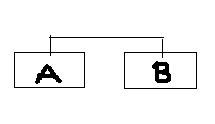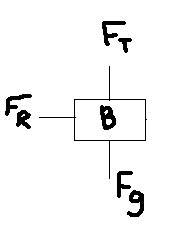# Free body diagram?

## Homework Statement

Two boxes of masses m=35kg and m2=45kg, are hung vertically from opposite ends of a rope passing over a rigid horizontal metal rod. They system starts moving from rest. Assuming that friction between the rod and the rope is negligible, determine the magnitude of
(a) the acceleration of the boxes
(b) the tension in the rope
(c) the magnitude of each box's displacement after 0.5s

## Homework Equations

Fnet=ma
Fnet= FT - Fg
ma=FT-Fg

(well i think this is right, depending on how the free body diagram is drawn..)

## The Attempt at a Solution

Well...I have no idea what the question is saying, im unsure how to draw the actual diagram and also how to draw the FBD. This is how i inteperted it:maybe im reading it wrong, but the way i read it was that there are 2 boxes tied to each other with a rope and that rope is hung on an iron bar.

hage567
Homework Helper
What's F_R in your diagram?? I agree with fizz on the interpretation of the problem.

Doc Al
Mentor
I assume you are to treat this just as you would if the rod were a frictionless (and massless) pulley. Draw a free body diagram for each mass separately.

Apply Newton's 2nd law to each mass (just like in your equations) to get two equations, which you'll solve together. Hint: Adopt a consistent sign convention, for example: up is positive and down is negative. Let "a" be the magnitude of the acceleration; how do the accelerations of the masses relate to each other?